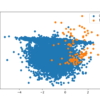# Archive | Imbalanced Classification## A Gentle Introduction to the Fbeta-Measure for Machine Learning

Fbeta-measure is a configurable single-score metric for evaluating a binary classification model based on the predictions made for the positive class. The Fbeta-measure is calculated using precision and recall. Precision is a metric that calculates the percentage of correct predictions for the positive class. Recall calculates the percentage of correct predictions for the positive class […]## How to Develop an Imbalanced Classification Model to Detect Oil Spills

Many imbalanced classification tasks require a skillful model that predicts a crisp class label, where both classes are equally important. An example of an imbalanced classification problem where a class label is required and both classes are equally important is the detection of oil spills or slicks in satellite images. The detection of a spill […]## How to Develop a Probabilistic Model of Breast Cancer Patient Survival

Developing a probabilistic model is challenging in general, although it is made more so when there is skew in the distribution of cases, referred to as an imbalanced dataset. The Haberman Dataset describes the five year or greater survival of breast cancer patient patients in the 1950s and 1960s and mostly contains patients that survive. […]## Why Is Imbalanced Classification Difficult?

Imbalanced classification is primarily challenging as a predictive modeling task because of the severely skewed class distribution. This is the cause for poor performance with traditional machine learning models and evaluation metrics that assume a balanced class distribution. Nevertheless, there are additional properties of a classification dataset that are not only challenging for predictive modeling […]## One-Class Classification Algorithms for Imbalanced Datasets

Outliers or anomalies are rare examples that do not fit in with the rest of the data. Identifying outliers in data is referred to as outlier or anomaly detection and a subfield of machine learning focused on this problem is referred to as one-class classification. These are unsupervised learning algorithms that attempt to model “normal” […]## Bagging and Random Forest for Imbalanced Classification

Bagging is an ensemble algorithm that fits multiple models on different subsets of a training dataset, then combines the predictions from all models. Random forest is an extension of bagging that also randomly selects subsets of features used in each data sample. Both bagging and random forests have proven effective on a wide range of […]## A Gentle Introduction to Threshold-Moving for Imbalanced Classification

Classification predictive modeling typically involves predicting a class label. Nevertheless, many machine learning algorithms are capable of predicting a probability or scoring of class membership, and this must be interpreted before it can be mapped to a crisp class label. This is achieved by using a threshold, such as 0.5, where all values equal or […]## Cost-Sensitive Learning for Imbalanced Classification

Most machine learning algorithms assume that all misclassification errors made by a model are equal. This is often not the case for imbalanced classification problems where missing a positive or minority class case is worse than incorrectly classifying an example from the negative or majority class. There are many real-world examples, such as detecting spam […]## How to Configure XGBoost for Imbalanced Classification

The XGBoost algorithm is effective for a wide range of regression and classification predictive modeling problems. It is an efficient implementation of the stochastic gradient boosting algorithm and offers a range of hyperparameters that give fine-grained control over the model training procedure. Although the algorithm performs well in general, even on imbalanced classification datasets, it […]## How to Develop a Cost-Sensitive Neural Network for Imbalanced Classification

Deep learning neural networks are a flexible class of machine learning algorithms that perform well on a wide range of problems. Neural networks are trained using the backpropagation of error algorithm that involves calculating errors made by the model on the training dataset and updating the model weights in proportion to those errors. The limitation […]